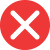calulate

Incorrect spelling, explanation: if we take the word calulate apart, we end up with the base word calul(us) and a verb suffix -ate. As you may know, there is no such word as calul or calulus, one c is definitely missing here, and when pronounced it doesn’t sound right. Calculate, on the other hand, is based on the Latin word calculus, and it makes total sense, both in meaning and pronunciation.calculate

Correct spelling, explanation: calculate is a verb made from the Latin-based word calculus. In order to create a verb out of a noun, the verb-creating suffix -ate is added in place of the suffix -us. Besides that, the pronunciation is changed, but the spelling remains relatively similar to the original.

Definition of calculate:
verb
1. To judge the number or amount of something by using mathematical methods
There are special formulas to calculate huge distances in the universe.
I’ll let you know when I calculate how long it will take me to get home.caluculate

Incorrect spellingcaluclate

Incorrect spellingcallculate

Incorrect spelling